Home

### Cycles and subschemes (14Cxx)

• Article
• ##### Noninjectivity of the cycle class map in continuous$\ell$ -adic cohomology
• Forum of Mathematics, Sigma, Volume 11
• Article
• ##### The integral Chow ring of$\mathcal {M}_{0}(\mathbb {P}^r, d)$, for$d$ odd
• Compositio Mathematica, Volume 159, Issue 1

### Families, fibrations (14Dxx)

• Article
• ##### The integral Chow ring of$\mathcal {M}_{0}(\mathbb {P}^r, d)$, for$d$ odd
• Compositio Mathematica, Volume 159, Issue 1

• Article
• ##### A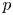$p$-adic monodromy theorem for de Rham local systems
• Compositio Mathematica, Volume 158, Issue 12

### (Co)homology theory (14Fxx)

• Article
• ##### Noninjectivity of the cycle class map in continuous$\ell$ -adic cohomology
• Forum of Mathematics, Sigma, Volume 11

• Article
• ##### COHOMOLOGY OF THE BRUHAT–TITS STRATA IN THE UNRAMIFIED UNITARY RAPOPORT–ZINK SPACE OF SIGNATURE$(1,n-1)$
• Nagoya Mathematical Journal, First View

### Arithmetic problems. Diophantine geometry (14Gxx)

• Article
• ##### A$p$-adic monodromy theorem for de Rham local systems
• Compositio Mathematica, Volume 158, Issue 12

### Curves (14Hxx)

• Article
• ##### The integral Chow ring of$\mathcal {M}_{0}(\mathbb {P}^r, d)$, for$d$ odd
• Compositio Mathematica, Volume 159, Issue 1

• Article
• #####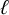$\ell$ -adic images of Galois for elliptic curves over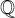$\mathbb {Q}$ (and an appendix with John Voight)
• Forum of Mathematics, Sigma, Volume 10

### Abelian varieties and schemes (14Kxx)

• Article
• ##### Torsion codimension$2$ cycles on supersingular abelian varieties
• Canadian Mathematical Bulletin, First View

### Algebraic groups (14Lxx)

• Article
• ##### COHOMOLOGY OF THE BRUHAT–TITS STRATA IN THE UNRAMIFIED UNITARY RAPOPORT–ZINK SPACE OF SIGNATURE$(1,n-1)$
• Nagoya Mathematical Journal, First View

• Article
• ##### ON$\mu _{n}$ -ACTIONS ON K3 SURFACES IN POSITIVE CHARACTERISTIC
• Nagoya Mathematical Journal, First View

### Affine geometry (14Rxx)

• Article
• ##### ON SEPARABLE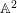$\mathbb{A}^{2}$ AND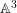$\mathbb{A}^{3}$-FORMS
• Nagoya Mathematical Journal, Volume 239